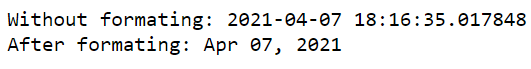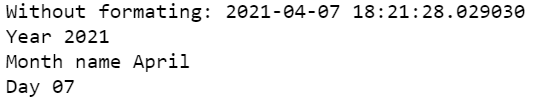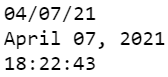# How to Format date using strftime() in Python ?

• Last Updated : 17 Apr, 2021

In this article, we will see how to format date using strftime() in Python. localtime() and gmtime() returns a tuple representing a time and this tuple is converted into a string as specified by the format argument using python time method strftime().

Syntax:

time.strftime(format[, sec])

sec: This is the time in number of seconds to be formatted.

format: This is the directive or format code which would be used to format.

Below are some examples for better understanding.

Example 1:

## Python3

 `from` `datetime ``import` `datetime`` ` `# current time and date``# datetime object``time ``=` `datetime.now()``print``(``"Without formating:"``, time)`` ` `# formating date using strftime``print``(``"After formating:"``, time.strftime(``"%b %d, %Y"``))`

Output:Example 2:

## Python3

 `from` `datetime ``import` `datetime`` ` `# current time and date``# datetime object``time ``=` `datetime.now()``print``(``"Without formating:"``, time)`` ` `# formating date using strftime``print``(``"Year"``, time.strftime(``"%Y"``))``print``(``"Month name"``, time.strftime(``"%B"``))``print``(``"Day"``, time.strftime(``"%d"``))`

Output:Example 3:

## Python3

 `from` `datetime ``import` `datetime`` ` `# current time and date``# datetime object``time ``=` `datetime.now()`` ` `# formating date using strftime``# format = MM/DD/YY``print``(time.strftime(``"%m/%d/%y"``))`` ` `# format = Month D, Yr``print``(time.strftime(``"%B %d, %Y"``))`` ` `# time formating``# HH:MM:SS``print``(time.strftime(``"%H:%M:%S"``))`

Output:My Personal Notes arrow_drop_up